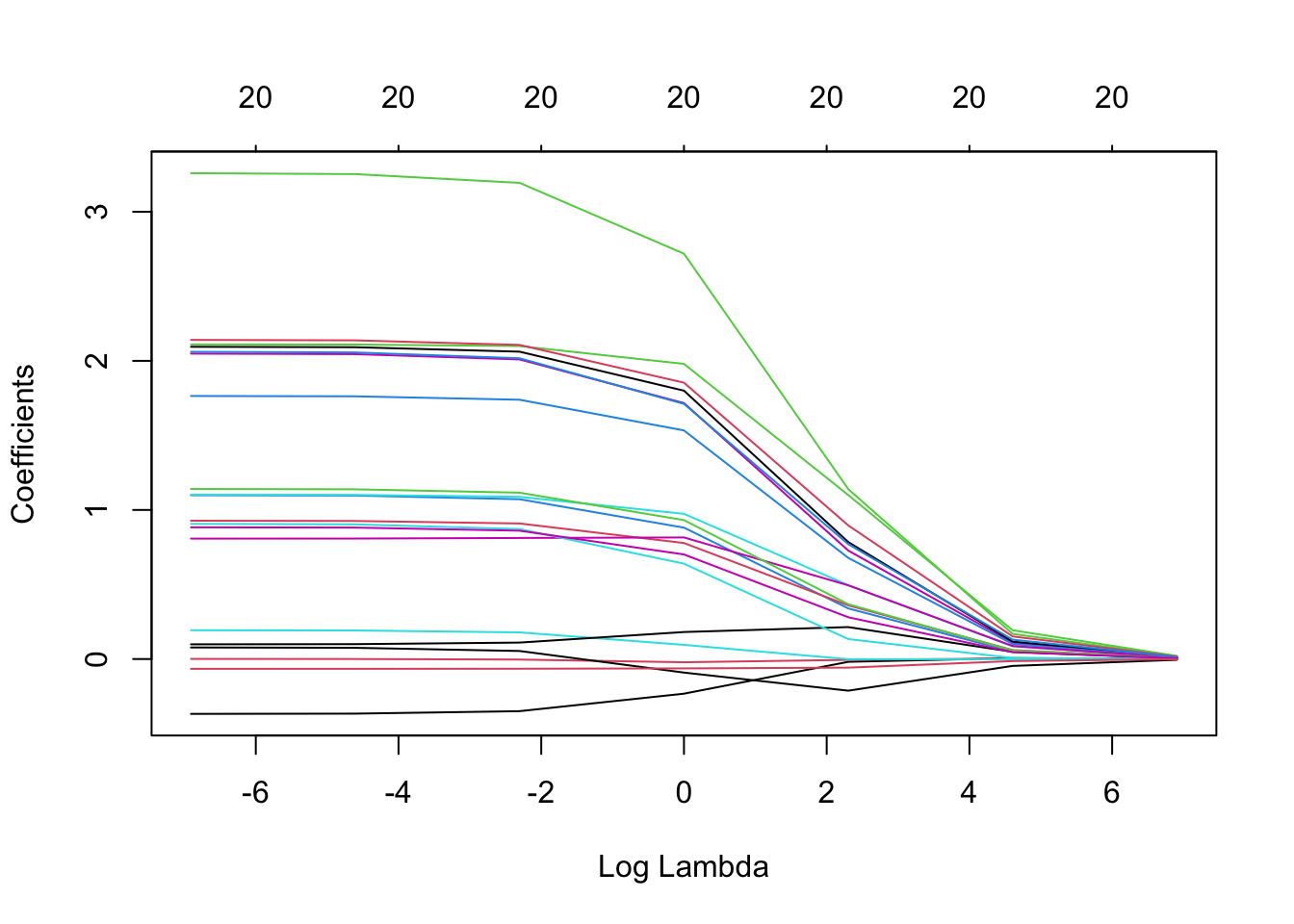knitr::opts_chunk\$set(echo = TRUE)
library(tidyverse)
library(broom)
# install.packages("glmnet") # if necessary
library(glmnet)

# High-dimensional regression

Generate some data from a high-dimensional model (increase p below)

#set.seed(1)
n <- 100
p <- 20
X <- matrix(rnorm(n*p), nrow = n)
beta <- rpois(p, lambda = 1)
#beta <- c(-1, 1, rep(0, p - 2))
y <- X %*% beta + rnorm(n)

## Ridge regression with glmnet

We will study ridge regression in more detail soon. For now you only need to know it’s a method we can use for fitting high-dimensional regression models. It also involves a tuning parameter called “lambda,” and larger values of lambda result in simpler fitted models. (We’ll learn about how they are simpler soon when we study penalized regression)

Use the glmnet package to fit a ridge regression model on the same data as in the previous part. Hint: read about the alpha input to the glmnet function in the documentation.

model_ridge <- glmnet(X, y,
intercept = FALSE,
alpha = 0,
lambda = 10^(-3:3))

What does plotting the model object show?

plot(model_ridge, xvar = "lambda")## Estimation error

Compute the mean-squared error of the coefficient estimates at different values of lambda

lambda <- 10^0
beta_hat <- coef(model_ridge, s = lambda)[-1] # leave out intercept
mean((beta - beta_hat)^2)
##  0.04761273

## Prediction error

Compute predictions using the estimated coefficients and the mean-squared prediction error

lambda <- 10^0
y_hat <- X %*% beta_hat
mean((y - y_hat)^2)
##  1.574581

# Overfitting to variance and ID generalisation

Generate a new sample from the same distribution (which things stay fixed?) and calculate the prediction error on this new sample at different values of lambda

X_ID <- matrix(rnorm(n*p), nrow = n)
y_ID <- X_ID %*% beta + rnorm(n)
y_ID_hat <- predict(model_ridge, newx = X_ID, s = lambda)
mean((y_ID - y_ID_hat)^2)
##  2.400369
y_hat <- predict(model_ridge, newx = X, s = 10*lambda)
mean((y - y_hat)^2)
##  15.74938

# “Overfitting to bias” and OOD generalisation

There are many ways to change the distribution for a new sample

• Change beta (maybe add a small amount of noise)
• Change the CEF some other way (e.g. add a non-linear term)
• Change the distribution of X and/or the errors

Pick one of these and test OOD generalisation (prediction error on new, different sample)

beta_new_D <- beta + rnorm(p, sd = .1)
X_OOD <- matrix(rnorm(n*p), nrow = n)
y_OOD <- X_OOD %*% beta_new_D + rnorm(n)
y_OOD_hat <- predict(model_ridge, newx = X_OOD, s = 10*lambda)
mean((y_OOD - y_OOD_hat)^2)
##  14.1588

## Discussion

#### How do these compare if we increase the original (training) sample size?

Copy/paste your gradient descent (or stochastic version) code for ordinary linear regression here. Does it converge? If so, to what? Check distance (MSE) from the true beta and from the ridge estimate of beta (at different values of lambda, or a very small lambda close to zero)

(Also consider generating data with p larger than n, so the least squares estimator is undefined)

# Gradient of the loss function
least_squares_gradient <- function(x, y, beta) {
-2 * t(x) %*% (y - x %*% beta) #+ 2 * beta
}

# Loss function
least_squares_loss <- function(x, y, beta) {
sum((y - x %*% beta)^2)
}

beta_prev2 <- rep(0, p) # or random start point
previous_loss <- least_squares_loss(X, y, beta_prev2)
next_loss <- least_squares_loss(X, y, beta_prev1)
steps <- 1

while (abs(previous_loss - next_loss) > 0.001) {

beta_prev2 <- beta_prev1
beta_prev1 <- beta_prev1 - step_BB * grad_prev1

previous_loss <- next_loss
next_loss <- least_squares_loss(X, y, beta_prev1)

#if (round(steps/10) == steps/10)
print(previous_loss)
steps <- steps + 1
}
##  4442.796
##  443.3899
##  156.6641
##  106.5216
##  89.82202
##  81.04247
##  77.52502
##  77.01596
##  76.54723
##  76.48063
##  76.4059
##  76.37042
beta_final <- beta_prev1 
mean((beta_final - beta)^2)
##  0.02336401
beta_zero <- coef(model_ridge, s = 10^(-3))[-1]
mean((beta_final - beta_zero)^2)
##  3.683847e-07